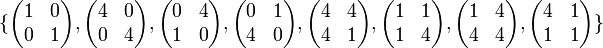# 2-Sylow subgroup of special linear group:SL(2,5)

This article is about a particular subgroup in a group, up to equivalence of subgroups (i.e., an isomorphism of groups that induces the corresponding isomorphism of subgroups). The subgroup is (up to isomorphism) quaternion group and the group is (up to isomorphism) special linear group:SL(2,5) (see subgroup structure of special linear group:SL(2,5)).
VIEW: Group-subgroup pairs with the same subgroup part | Group-subgroup pairs with the same group part | All pages on particular subgroups in groups$G$ is the special linear group:SL(2,5), i.e., the special linear group of degree two over field:F5. In other words, it is the group of invertible$2 \times 2$ matrices of determinant 1 over the field with three elements. The field has elements 0,1,2,3,4 with$4 = -1$.$H$ is the subgroup:$\{ \begin{pmatrix} 1 & 0 \\ 0 & 1 \\\end{pmatrix}, \begin{pmatrix} 4 & 0 \\ 0 & 4 \\\end{pmatrix}, \begin{pmatrix} 0 & 4 \\ 1 & 0 \\\end{pmatrix}, \begin{pmatrix} 0 & 1 \\ 4 & 0 \\\end{pmatrix}, \begin{pmatrix} 4 & 4 \\ 4 & 1 \\\end{pmatrix}, \begin{pmatrix} 1 & 1 \\ 1 & 4 \\\end{pmatrix}, \begin{pmatrix} 1 & 4 \\ 4 & 4 \\\end{pmatrix}, \begin{pmatrix} 4 & 1 \\ 1 & 1 \\\end{pmatrix} \}$$H$ is isomorphic to the quaternion group of order 8.

## Arithmetic functions

Function Value Explanation
order of the whole group 120 Order of$SL(2,q)$ is$q^3 - q$. Here$q = 5$.
order of the subgroup 8
index of the subgroup 15
size of conjugacy class = index of normalizer 5
number of conjugacy classes in automorphism class 1